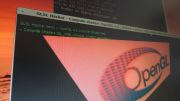Home Utilities Tutorials 3D Demos Graphics Cards Forums About

 GeeXLab
Current version: 0.29.17
>GeeXLab homepage

 FurMark
Current version: 1.21.1
>FurMark homepage

 GPU Caps Viewer
Current version: 1.44.2.1
>GPU Caps Viewer homepage

 GPU Shark
Current version: 0.16.1.0
>GPU Shark homepage

 Blogs
>JeGX's HackLab

 Geeks3D's Articles
>GPU Memory Speed Demystified

>How to Get your Multi-core CPU Busy at 100%

>How To Make a VGA Dummy Plug

>Night Vision Post Processing Filter

 PhysX FluidMark
Current version: 1.5.4
>FluidMark homepage

 TessMark
Current version: 0.3.0
>TessMark homepage

Current version: 0.3.0

 Demoniak3D
Current Version: 1.23.0
>Demoniak3D
>Libraries and Plugins
>Demos
>Codes Samples

3D Graphics Search Engine:Fog in GLSL By Jerome 'JeGX' Guinot - jegx_AT_ozone3d(dot)net Initial draft: December 21, 2007 [ Index ]»Next Page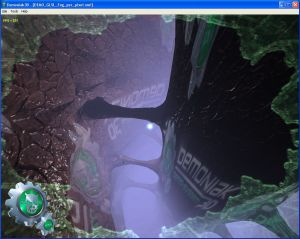4 - Per-Pixel Fog ComputationWe shall see here how to compute the fog at each pixel level . The principle is exactly the same as by vertex. The only difference resides in the determination of the distance between the camera and the currently processed pixel . In the per-pixel fog computation, the vertex shader is inactive, because the calculus is made in the pixel shader . In GLSL there exists a gl_FragCoord variable. This one is an input variable of the pixel shader. gl_FragCoord contains the screen coordinates of the current pixel. For example, if the demo's window has a width of 1280 pixels, the following code : ```if(gl_FragCoord.x < 700.0) { gl_FragColor = vec4(0.0, 0.5, 0.0, 1.0); } else { gl_FragColor = mix(gl_Fog.color, finalColor, fogFactor ); }``` should give the following image :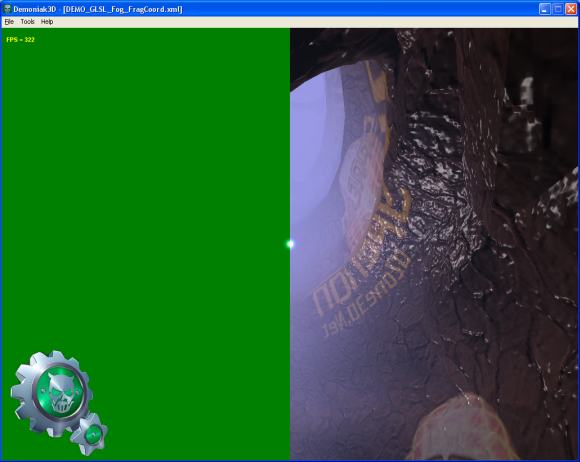DEMO_GLSL_Fog_FragCoord.xml The distance between the camera and the current pixel (the z axis value) is obtained by the following relation : `float z = gl_FragCoord.z / gl_FragCoord.w;` It is quite easy, thanks to this relation, to show a z-buffer view from the camera's point of view: ```float z = 1.0 - (gl_FragCoord.z / gl_FragCoord.w) / 4000.0; gl_FragColor = vec4(z, z, z, 1.0);```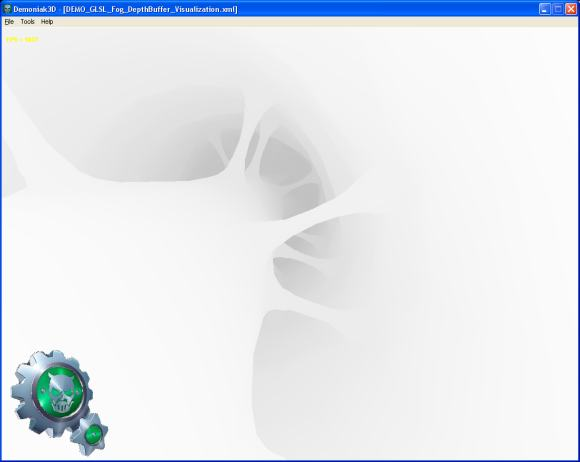DEMO_GLSL_Fog_DepthBuffer_Visualization.xml The 4000.0 corresponds to the far clipping plane (zfar), initialized in the Demoniak3D demo. That said, let's come back to the fog computation. The following code show how to implement the fog calculus per-pixel: ```const float LOG2 = 1.442695; float z = gl_FragCoord.z / gl_FragCoord.w; float fogFactor = exp2( -gl_Fog.density * gl_Fog.density * z * z * LOG2 ); fogFactor = clamp(fogFactor, 0.0, 1.0); gl_FragColor = mix(gl_Fog.color, finalColor, fogFactor );``` [ Index ]»Next Page

 GeeXLab demosGLSL - Mesh exploderPhysX 3 cloth demo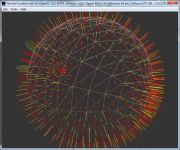Normal visualizer with GS